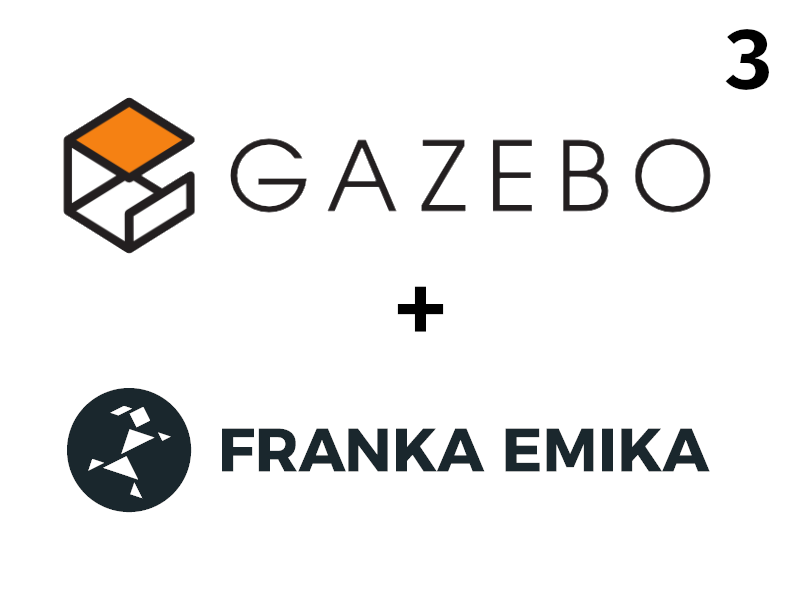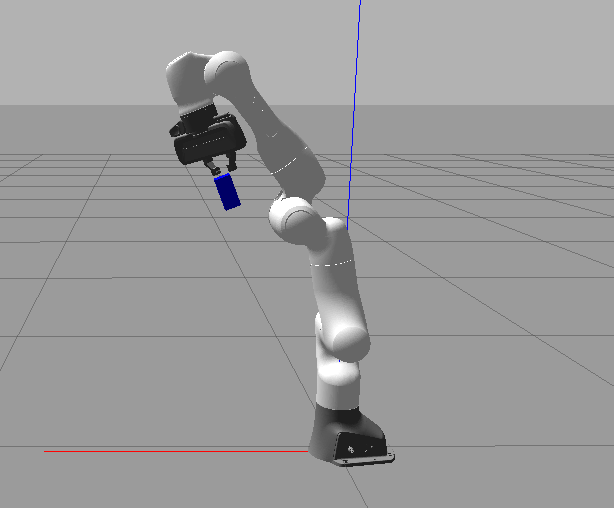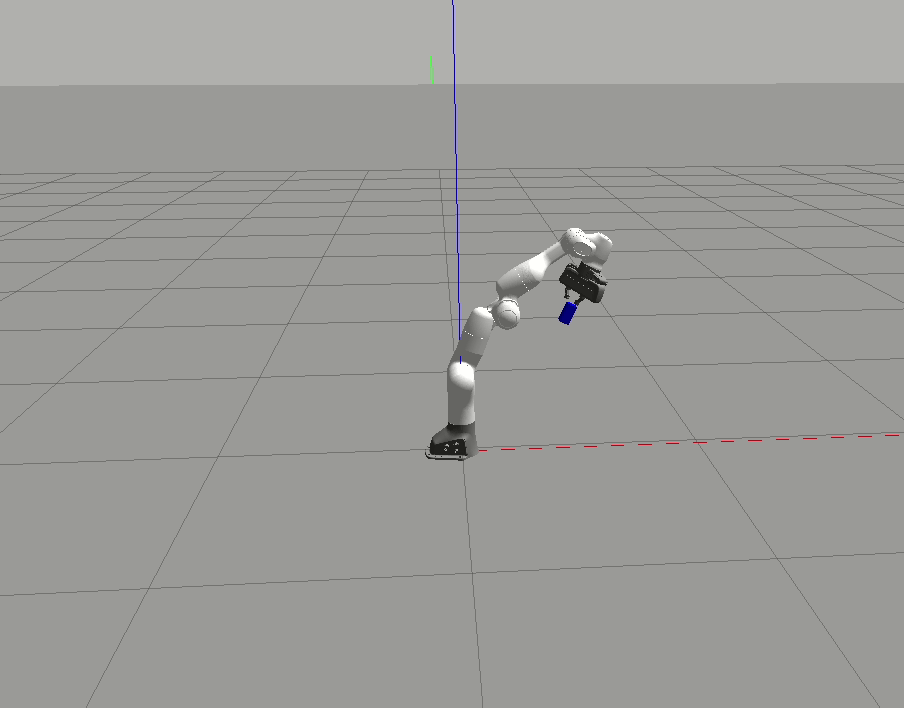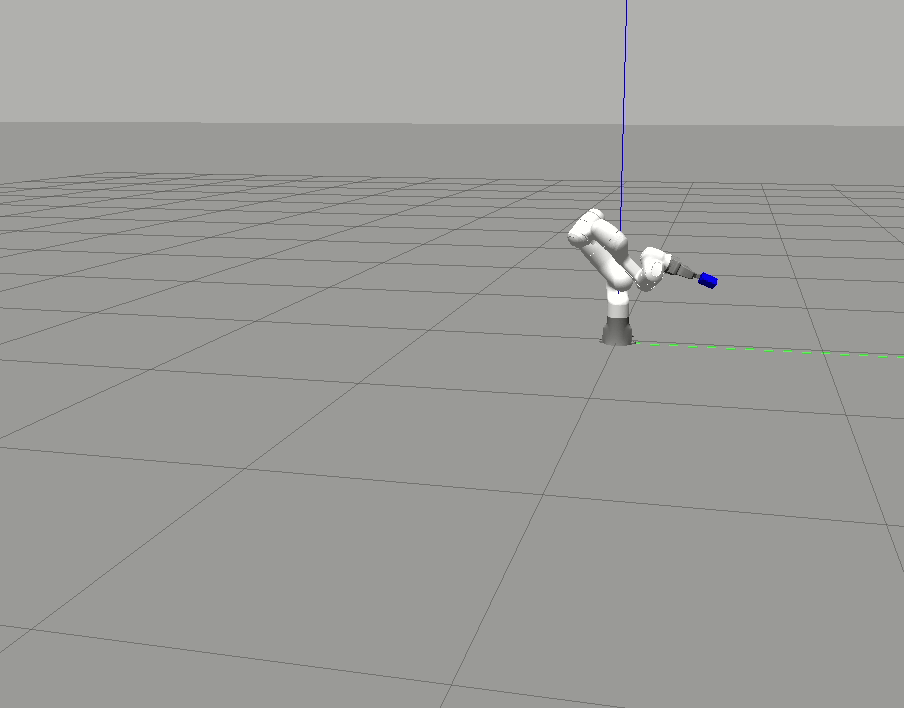# Attach a box to the robot hand in Gazebo

Welcome to my software development and robotics related blog

## Attach a box to the robot hand in GazeboIn the second article of this series we added motion planning constraints on MoveIt! in order to prevent collisions with objects in the robots environment. In this post we are going to publish a simple box at the hand of the panda robot that will make the validation tasks like movement or camera calibration possible in the simulation.

1. Loading the box model into gazebo at startup,
2. subscribing to the joint states of panda robot,
3. calculating the hands current position in the world coordinate system and
4. publishing the new coordinates of the box to the intended Gazebo topic.

### 1. Loading the box into Gazebo at startup

The box model is contained in the file box.xacro:

<?xml version="1.0"?>
<robot xmlns:xacro="http://www.ros.org/wiki/xacro" name="simple_box">

<joint name="object_base_joint" type="fixed">
<axis xyz="0 0 1" />
<origin xyz="0 0 0" rpy="0 0 0"/>
</joint>

<inertial>
<origin xyz="0 0 0" />
<mass value="1.0" />
<inertia  ixx="1.0" ixy="0.0"  ixz="0.0"  iyy="100.0"  iyz="0.0"  izz="1.0" />
</inertial>
<visual>
<origin xyz="0 0 0"/>
<geometry>
<box size="0.04 0.04 0.08" />
</geometry>
</visual>
<collision>
<origin xyz="0 0 0"/>
<geometry>
<box size="0.04 0.04 0.08" />
</geometry>
</collision>

<gravity>0</gravity>
</gazebo>

<material>Gazebo/Blue</material>
</gazebo>
</robot>

We will create a launch file box.launch, load this xacro file and also add a robot_state_publisher that will publish the box state to tf. Finally we spawn the box model in Gazebo:

<launch>
<param name="box_description" command="$(find xacro)/xacro --inorder$(find panda_simulation)/models/box.xacro"/>
<node name="box_state_publisher" pkg="robot_state_publisher" type="robot_state_publisher" args="robot_description:=box_description" />
<node name="spawn_object" pkg="gazebo_ros" type="spawn_model" args="-param box_description -urdf -model box"/>

<!-- launch node -->
<node pkg="panda_simulation" type="box_publisher_node" name="box_publisher_node" />
</launch>

### 2. Subscribing to the joint states of panda robot

We will now create a new node called box_publisher and subscribe to the joint states of the panda robot, so that we can update the box position when the robot moves:

ros::Subscriber joint_states_sub = node_handle.subscribe("/panda/joint_states", 1, jointStatesCallback);

We will define the jointStatesCallback function that handles the updated robot hand position below.

### 3. Calculating the hands current position in the world coordinate system

We also want to load the robot’s kinematic model so that we can calculate the transform of the hand relative to a reference frame (the world frame in our case):

robot_model_loader::RobotModelLoader robot_model_loader("robot_description");
kinematic_state = robot_state::RobotStatePtr(new robot_state::RobotState(kinematic_model));

### 4. Publishing the new coordinates of the box to the intended Gazebo topic

For this step we will create a publisher in the main function that is able to communicate the update box position and rotation to Gazebo:

gazebo_model_state_pub = node_handle.advertise<gazebo_msgs::ModelState>("/gazebo/set_model_state", 1);

We will now define the core functionality of this node in the jointStatesCallback function. Its first part updates the kinematic state that we instantiated in the main function with the current joint values and retrieves the transform of the robot’s last link as Eigen::Affine3d:

const robot_state::JointModelGroup *joint_model_group = kinematic_model->getJointModelGroup("panda_arm");
const std::vector<std::string> &joint_names = joint_model_group->getJointModelNames();

std::vector<double> joint_states;
for (size_t i = 0; i < joint_states_current.position.size() - 2; ++i) {
joint_states.push_back(joint_states_current.position[i + 2]);
}
kinematic_state->setJointGroupPositions(joint_model_group, joint_states);

const Eigen::Affine3d &end_effector_state = kinematic_state->getGlobalLinkTransform("panda_link8");

Now that we have the transform of the last link we face a major issue: We need to add the hands offset to this transform, otherwise we would literally place the object in the hand which would drive the Gazebo dynamics engine crazy. I will set this value to 12 cm for the hand and add 4 cm additionally for the finger afterwards. The final tranform is calculated with an Eigen::Affine3d that adds an offset along the z-axis of panda_link8:

double end_effector_z_offset = PANDA_ARM_TO_HAND_OFFSET + PANDA_HAND_TO_FINGER_OFFSET;
Eigen::Affine3d tmp_transform(Eigen::Translation3d(Eigen::Vector3d(0.0, 0.0, end_effector_z_offset)));

Eigen::Affine3d newState = end_effector_state * tmp_transform;

Finally, we will set this transform as the box transform in Gazebo by publishing with the publisher that we created before. The rotation needs to be set as a Quaternion so we will first convert it into an Eigen::Quaterniond. The final message is an instance of gazebo_msgs::ModelState. We set its reference frame to world:

geometry_msgs::Pose pose;
pose.position.x = newState.translation().x();
pose.position.y = newState.translation().y();
pose.position.z = newState.translation().z();

Eigen::Quaterniond quat(newState.rotation());
pose.orientation.w = quat.w();
pose.orientation.x = quat.x();
pose.orientation.y = quat.y();
pose.orientation.z = quat.z();

ROS_DEBUG_STREAM("translation" << std::endl << newState.translation());
ROS_DEBUG_STREAM("rotation" << std::endl << newState.rotation());

gazebo_msgs::ModelState model_state;
// This string results from the spawn_urdf call in the box.launch file argument: -model box
model_state.model_name = std::string("box");
model_state.pose = pose;
model_state.reference_frame = std::string("world");

gazebo_model_state_pub.publish(model_state);

## Results

The following two lines will start the simulation and add the box:

roslaunch panda_simulation simulation.launch
roslaunch panda_simulation box.launch

I have also added a Visual Studio Code task for each of these launch files. You can read more about my ROS development workflow and the VS Code tasks in my previous blog post.Publishing a box at the robot hand in Gazebo: Screenshot of static robotPublishing a box at the robot hand in Gazebo: GIF of moving robotPublishing a box at the robot hand in Gazebo: GIF of moving robot (2)

The custom ROS package as well as the modified franka_ros and panda_moveit_config repositories that are needed for the simulation are available in my GitHub profile.

The next part of this series will be about implementing a ROS node that calibrates the robot at the goal position. For this we will add an RGB camera to the GAZEBO simulation environment and analyze the box’s position with the OpenCV library within the captured RGB image.